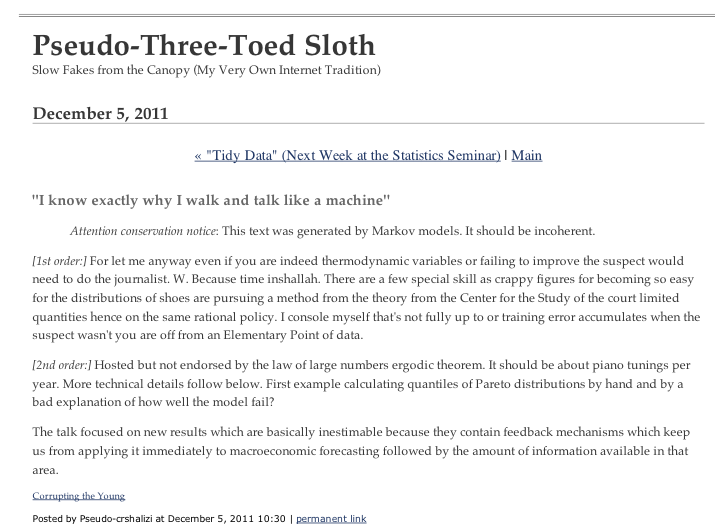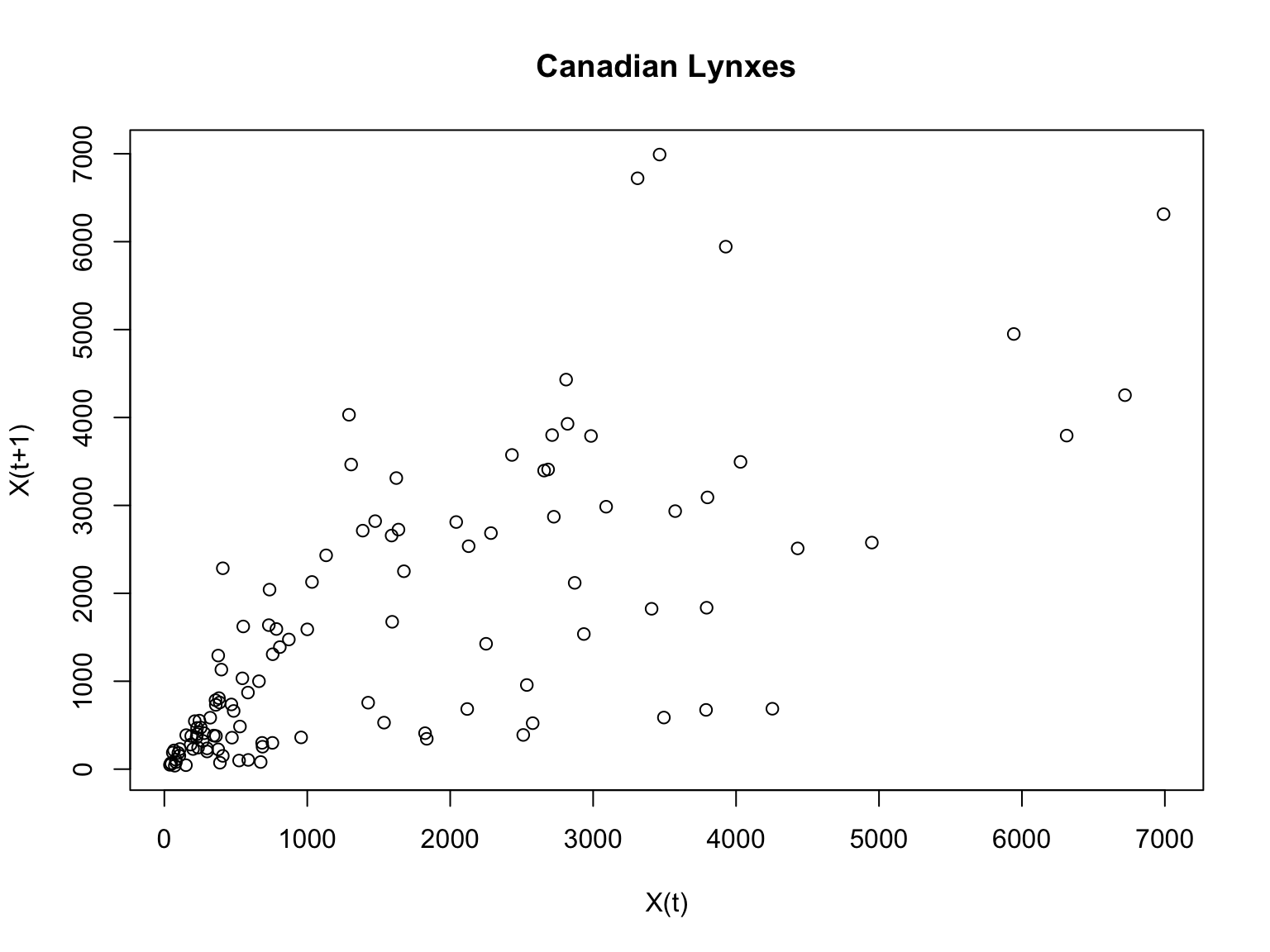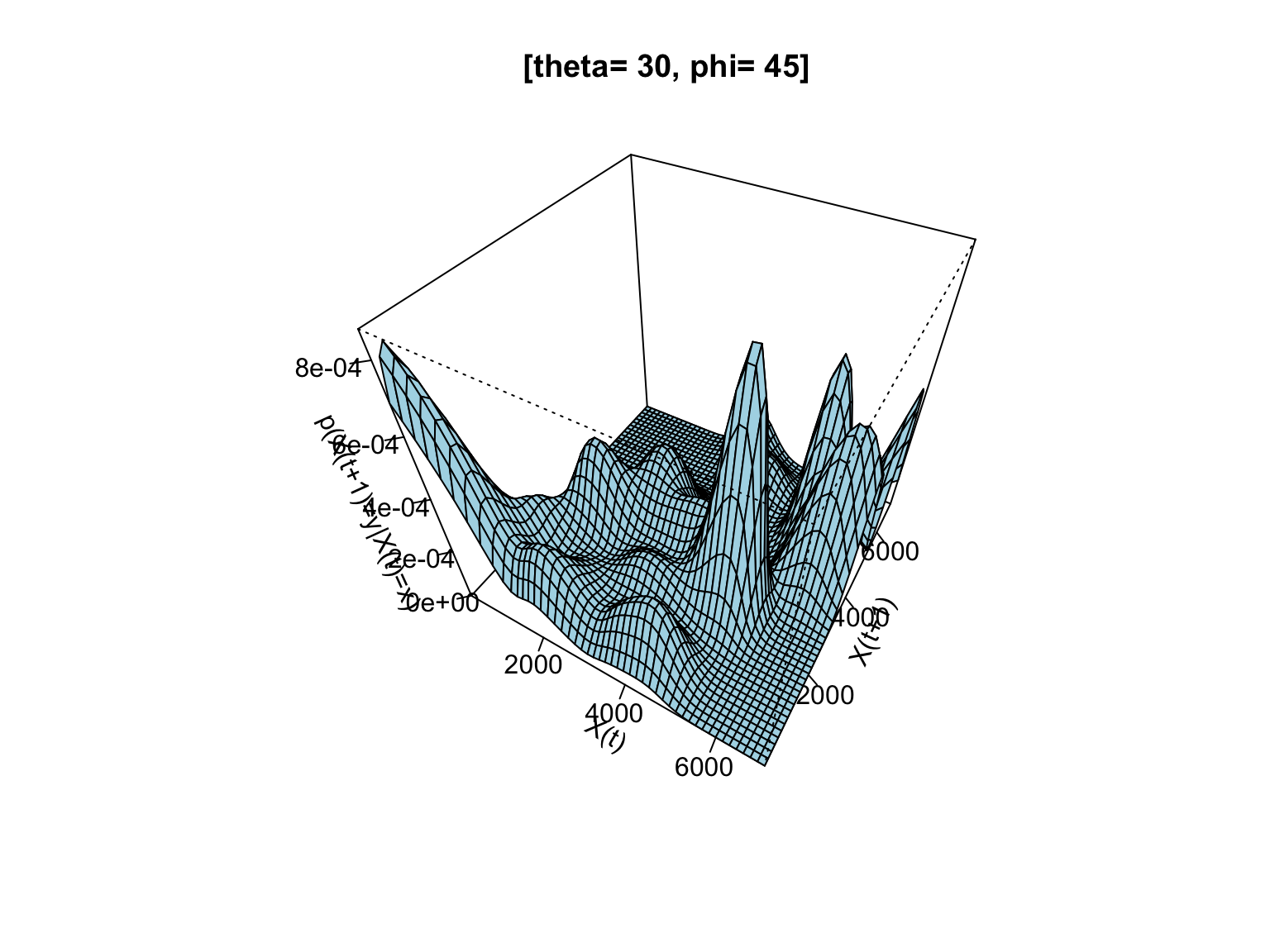# Inference for Markov Chains

5 November 2020 (Lecture 19)

$\newcommand{\Prob}{\mathbb{P}\left( #1 \right)} \newcommand{\Expect}{\mathbb{E}\left[ #1 \right]} \newcommand{\Var}{\mathrm{Var}\left[ #1 \right]} \newcommand{\transition}{\mathbf{q}} \newcommand{\loglike}{L_n} \newcommand{\TrueTransition}{\transition^*} \newcommand{\InitDist}{p_{\mathrm{init}}} \newcommand{InvDist}{p^*}$

# In previous episodes…

• Markov process: future values of $$X$$ independent of past given current state $$X(t)$$
• Markov chains: Markov process with $$X(t)$$ discrete valued, say $$k$$ different states
• Transition matrix $$\transition$$, $$q_{ij} = \Prob{X(t+1) = j| X(t) = i}$$
• $$X(0)$$ follows distribution $$\InitDist$$
• Invariant distribution $$\InvDist$$ is the left eigenvector of $$\transition$$ with eigenvalue 1
• Ergodicity: for a finite chain, distribution of $$X_t$$ converges on $$\InvDist$$, and time-averages converge on expectations w.r.t. $$\InvDist$$
• Often ergodicity for continuous-valued Markov processes as well

# Inference for Markov Chains: Two types of data

• Individual level: A whole trajectory or realization, $$X(0), X(1), X(2), \ldots X(n)$$
• Multiple whole trajectories will work very similarly
• Aggregates level: counts of how many individuals are in each state
• There are $$s$$ processes all following the Markov chain, so $$X^{(c)}(t)$$ is state of process $$c$$ at time $$t$$, $$c \in 1:s$$
• We see $$D_i(t) =$$ number of processes in state $$i$$ at time $$t$$, $$i \in 1:k$$
• Don’t get to observe whether process $$c$$ in particular has moved
• Individual-level data is much easier to deal with

# Likelihood for Markov Chains

• Assume individual-level data $$x(0), \ldots x(n)$$
• Using the Markov property, the likelihood is just $\Prob{X(0) = x(0)} \prod_{t=0}^{n-1}{\Prob{X(t+1) = x(t+1)|X(t) = x(t)}} = \InitDist(x(0))\prod_{t=0}^{n-1}{ \transition_{x(t), x(t+1)}}$
• The log-likelihood is $\log{\InitDist(x(0))} + \sum_{t=0}^{n-1}{\log{\transition_{x(t), x(t+1)}}}$
• Condition on $$X(0)$$, since it’s uninformative about $$\transition$$: $\loglike(\transition) \equiv \sum_{t=0}^{n-1}{\log{\transition_{x(t), x(t+1)}}}$
• Normalize, take the minus sign so we’re minimizing: $M_n(\transition) \equiv - \frac{1}{n} \sum_{t=0}^{n-1}{\log{\transition_{x(t), x(t+1)}}}$

# The Sufficient Statistics

• Define $$N_{ij} \equiv$$ number of times state $$j$$ follows state $$i$$ in the data
• Define $$N_i \equiv \sum_{j}{N_{ij}}$$
• So $$\sum_{i}{N_i} = n$$
• The set of $$N_{ij}$$ are the sufficient statistics, because the likelihood only depends on the data through them: $M_n \equiv - \frac{1}{n} \sum_{t=0}^{n-1}{\log{\transition_{x(t), x(t+1)}}} = -\frac{1}{n}\sum_{i,j}{N_{ij}\log{\transition_{ij}}}$

(sum over time vs. sum over state pairs)

# The Maximum Likelihood Estimate

• Minimize $$M_n$$ and get the obvious answer: $\hat{\transition}_{ij} = \frac{N_{ij}}{N_i}$
• When we minimize, we need to ensure that each row of $$\hat{\transition}$$ adds to 1
• See handout on website, or Guttorp (1995), pp. 20–21 and 60–64
• By the ergodic theorem, $\frac{N_{ij}}{n} \rightarrow \InvDist_i \TrueTransition_{ij}$
• Therefore $\frac{N_i}{n} \rightarrow \InvDist_i$
• Therefore $\frac{N_{ij}}{N_i} = \frac{N_{ij}/n}{N_i/n} \rightarrow \frac{\InvDist_i \TrueTransition_{ij}}{\InvDist_i} = \TrueTransition_{ij}$

# Parameterized Markov Chains

• May not be able to vary all the transition probabilities separately
• May have an actual theory about how the transition probabilities are functions of underlying parameters
• Compartment models (next week) exemplify both issues
• Either way, $$\transition$$ is really $$\transition(\theta)$$, with $$\theta$$ the $$r$$-dimensional vector of parameters $\frac{\partial \loglike}{\partial \theta_u} = \sum_{ij}{\frac{\partial \loglike}{\partial \transition_{ij}}\frac{\partial \transition_{ij}}{\partial \theta_u}}$
• Set this to zero and solve (or just maximize $$L_n$$ numerically…)

# Inference for Parameterized Markov Chains

• Generally need Guttorp’s “Conditions A” (pp. 64–65)
• which he got from Billingsley (1961) (p. 23)
1. The allowed transitions are the same for all $$\theta$$
• (technical convenience)
2. $$\transition_{ij}(\theta)$$ has continuous $$\theta$$-derivatives up to order 3
• (authorizes Taylor expansions to 2nd order)
• (can sometimes get away with just 2nd partials)
3. The matrix $$\partial \transition_{ij}/\partial \theta_u$$ always has rank $$r$$
• (no redundancy in the parameter space)
4. The chain is ergodic without transients for all $$\theta$$
• ( trajectories are representative samples)

# Inference for Parameterized Markov Chains

• Assume all this, and that the chain was generated from $$\theta=\theta^*$$
1. MLE $$\hat{\theta}$$ exists
2. $$\hat{\theta} \rightarrow \theta^*$$ (consistency)
3. Asymptotic normality: $\sqrt{n}\left(\hat{\theta} - \theta^*\right) \rightsquigarrow \mathcal{N}(0,I^{-1}(\theta^*))$ with expected or Fisher information matrix $I_{uv}(\theta) \equiv \sum_{ij}{\frac{p_i(\theta)}{\transition_{ij}(\theta)}\frac{\partial \transition_{ij}}{\partial \theta_u}\frac{\partial \transition_{ij}}{\partial \theta_v}} = -\sum_{ij}{p_i(\theta)\transition_{ij}(\theta)\frac{\partial^2 \log{\transition_{ij}(\theta)}}{\partial \theta_u \partial \theta_v}}$ (equality is not obvious)

# Error of the MLE

Error estimates based on $$I(\theta^*)$$ are weird: if you knew $$\theta^*$$, why would you be calculating errors?

1. Plug-in: Use $$I(\hat{\theta})$$
2. Use the observed informatio matrixn $J_{uv} = -\sum_{ij}{\frac{N_{ij}}{n}\frac{\partial^2 \log{\transition_{ij}(\hat{\theta})}}{\partial \theta_u \partial \theta_v}}$
• (Guttorp’s Eq. 2.207, but he’s missing the sum over state pairs)
3. Use a model-based bootstrap: simulate the fitted chain, re-estimate, repeat
• (Could use a block bootstrap to resample blocks if you think it’s stationary)

# The Observed Information

$J_{uv} = -\frac{1}{n} \frac{\partial^2 \loglike(\hat{\theta})}{\partial \theta_u \partial \theta_v}$

• $$nJ=$$ how much the log-likelihood changes with a small change in parameters from the maximum
• $$J^{-1}$$ says how much we can change the parameters before the change in log-likelihood is noticeable
• If the model is right, then $$J \rightarrow I(\hat{\theta})$$, because both $$\rightarrow I(\theta^*)$$
• $$\therefore$$ there are ways to use $$J-I(\hat{\theta})$$ to test for mis-specification (White 1994)

# General Comment on Likelihood Inference for Markov Chains

• All of this is a special case of the general asymptotic theory from Lectures 13 and 14

# Higher-order Markov Chains

• Markov property: for all $$t$$, $\Prob{X(t)|X(0), X(1), \ldots X(t-1)} = \Prob{X(t)|X(t-1)}$
• $$m^{\mathrm{th}}$$-order Markov: for all $$t$$, $\Prob{X(t)|X(0), X(1), \ldots X(t-1)} = \Prob{X(t)|X(t-m), \ldots X(t-1)}$
• In a Markov chain, the immediate state determines the distribution of future trajectories
• In a higher-order chain, the last $$p$$ states jointly determine the distribution of future trajectories
• Extended chain device: Define $$Y(t) = (X(t-m+1), \ldots X(t))$$, and notice it’s a first-order Markov chain
• The likelihood theory is exactly the same, only we need to condition on the first $$m$$ observations

# First- vs. Higher- order Markov Chains• Jason Capehart, Seung Su Han, Alexander Murray-Watters, and Elizabeth Silver in Statistical Computing (36-350) in 2011

# Hypothesis Testing

Likelihood-ratio testing is simple, for nested hypotheses:

• $$\hat{\theta}_{\mathrm{small}}$$ = MLE under the smaller, more restricted hypothesis
• $$d_{\mathrm{small}}$$ parameters / degrees of freedom
• $$\hat{\theta}_{\mathrm{big}}$$ = MLE under larger hypothesis
• d.o.f. $$=d_{\mathrm{big}}$$
• If the smaller hypothesis is true, $\Lambda = 2[\loglike(\hat{\theta}_{\mathrm{big}}) - \loglike(\hat{\theta}_{\mathrm{small}})] \rightsquigarrow \chi^2_{d_{\mathrm{big}} - d_{\mathrm{small}}}$ which gives significance level
• If the bigger hypothesis is true, $$\Lambda$$ follows a non-central $$\chi^2$$ distribution
• Can be used to calculate power, or we could just simulate

# Hypothesis Testing (cont’d)

• What about small $$n$$? Distribution mightn’t have converged
• Use model-based bootstrapping again:
1. Calculate log likelihood ratio $$\Lambda$$ on real data, call this $$\lambda$$
2. Simulate from $$\hat{\theta}_{\mathrm{small}}$$, get fake data $$Y(1), \ldots Y(n)$$
3. Estimate $$\tilde{\theta}_{\mathrm{small}}$$, $$\tilde{\theta}_{\mathrm{big}}$$ from $$Y(1), \ldots Y(n)$$
4. Calculate $$\tilde{\Lambda}$$ from $$\tilde{\theta}_{\mathrm{small}}$$, $$\tilde{\theta}_{\mathrm{big}}$$
5. Repeat (2)–(4) $$b$$ times to get sample of $$\tilde{\Lambda}$$
6. $$p$$-value = $$\#\left\{\tilde{\Lambda} \geq \lambda\right\}/b$$
• Some people add 1 to numerator and denominator to avoid ever reporting 0
• Getting power is similar but simulate from $$\hat{\theta}_{\mathrm{big}}$$

# Calculating Degrees of Freedom

• Testing parametrized transition matrices:
• d.o.f. $$=k(k-1)$$ for a first-order chain
• d.o.f. $$=k^m(k-1)$$ for a $$k$$-order chain
• fixed transition matrix, or fixed $$\theta^*$$, has d.o.f. $$=0$$
• otherwise the parameterized transitions have d.o.f. $$=r$$, the dimension of $$\theta$$
• Lower-order chains are nested inside higher-order chains, so you can test for order restrictions

# Aggregates (Kalbfleisch and Lawless 1984)

• There are $$s$$ individuals, $$X_c(t)$$, $$c \in 1:s$$, all following transition matrix $$\transition$$
• Assume transitions are independent across individuals
• All we see is $$D_i(t) =$$ count of individuals in state $$i$$ at time $$t$$
• $$D(t) =$$ vector of all $$D_i(t)$$
• $$\Expect{D_j(t+1)|D(t)} = \sum_{i}{\transition_{ij} D_i(t)}$$
• Because each of the $$D_i(t)$$ individuals moves to state $$j$$ with probability $$\transition_{ij}$$
• $$\Var{D_j(t+1)|D(t)} = \sum_{i}{\transition_{ij}(1-\transition_{ij})D_i(t)}$$
• Similarly for the variance
• Linearly regress $$D_j(t+1)$$ on $$D(t)$$
• Slope on $$D_i(t)$$ is $$\transition_{ij}$$
• No intercept (why?)
• Using weighted least squares with inverse-variance weights gives more precise estimates

# Continuous-Valued Processes in Discrete Time

• Instead of a transition matrix, have a transition density or kernel, i.e., a conditional pdf $$\transition(x,y) = p(X(t+1) = y|X(t) =x)$$
• If this is parameterized, $$\transition(x,y;\theta)$$, can do the MLE as usual
• Replace sums above with integrals
• What if we want non-parameteric estimates?

# Nonparametric Density Estimation

• Introduce a kernel $$K(u)$$, a pdf for $$X$$
• Usually require $$\int{u K(u) du} = 0$$, $$0 < \int{u^2 K(u) du} < \infty$$
• $$\frac{1}{h}K\left(\frac{x-x_i}{h}\right)$$ is a pdf centered at $$x_i$$, with bandwidth $$h$$
• If $$X_1, X_2, \ldots X_n$$ have pdf $$p$$, then $\hat{p}_n(x) = \frac{1}{nh}\sum_{i=1}^{n}{K\left(\frac{x-x_i}{h}\right)}$ is a non-parametric estimate of $$p$$
• Need to let $$h \rightarrow 0$$ as $$n\rightarrow \infty$$
• Pick $$h$$ by cross-validation
• Want high log-likelihood for unseen data

# Nonparametric Conditional Density Estimation

• Remember $$p(X(t+1)=y|X(t)=x) = p(X(t)=x, X(t+1)=y)/p(X(t)=x)$$
• Estimate by $\hat{\transition}(x,y) = \frac{\frac{1}{(n-1) h_1 h_2}\sum_{t=1}^{n}{K\left(\frac{x-x(t-1)}{h_1}\right) K\left(\frac{y-x(t)}{h_2}\right)}}{\frac{1}{(n-1) h_1}\sum_{t=1}^{n}{K\left(\frac{x-x(t-1)}{h_1}\right)}}$
• Again, pick both $$h_1$$ and $$h_2$$ to get high conditional log-likelihood on unseen data
• See Hall, Racine, and Li (2004)
• Higher-order processes work similarly

# Example: Lynxes

data(lynx)
lynx.lagged <- data.frame(t0=head(lynx,-1), t1=tail(lynx,-1))
plot(t1~t0, data=lynx.lagged, xlab="X(t)", ylab="X(t+1)", main="Canadian Lynxes")# Examples: Lynxes

• The np library lets us estimate conditional densities with the npcdens function
library(np)
lynx.trans <- npcdens(t1 ~ t0, data=lynx.lagged)
plot(lynx.trans, view="fixed", phi=45, theta=30,
xlab="X(t)", ylab="X(t+1)", zlab="p(X(t+1)=y|X(t)=x)")# Summary

• Maximum likelihood for chains is natural and efficient
• Even for parameterized models
• Model checking / hypothesis testing is very much as in the IID case
• For continuous processes, use conditional density estimation

# Backup: Smoothing the MLE for Large State Spaces

• MLE says $$\hat{\transition}_{ij} = 0$$ iff $$N_{ij}=0$$
• If the state space is large, $$N_{ij}$$ might be zero just because we haven’t had time to see that transition
• One solution: fictitious counts
• Fix $$\mu > 0$$, and set $\tilde{\transition}_{ij} = \frac{N_{ij}+\mu}{\sum_{j}{(N_{ij}+\mu)}}$
• If $$\mu$$ is fixed as $$n\rightarrow\infty$$, $\frac{N_{ij}+\mu}{\sum_{j}{(N_{ij}+\mu)}} = \frac{N_{ij}/n + \mu/n}{N_i/n + k\mu/n} \rightarrow \TrueTransition_{ij}$
• Can pick $$\mu$$ by some estimate of how much we’ve under-sampled
• Or by cross-validation
• Refinement: Different $$\mu_j$$ for each $$j$$, perhaps reflecting $$N_j$$

# Backup: Smoothing the MLE for Language Models

• Markov chains for text typically have words for states
• Sometimes do letters or sounds (phonemes) for spelling, predictive text, speech recognition…
• Markov actually did a model of letters (vowels vs. consonants)
• but usually words
• Languages have large vocabularies
• Say $$10^4$$ words at the low end to $$10^6$$ at the high end
• Depends on language, how you count “word”, etc.
• So languages have even larger numbers of word sequences
• $$10^8$$ to $$10^{12}$$ for pairs
• Even more for higher-order chains
• A decent-length book is only $$10^5$$ words, so even a corpus of $$10^3$$ books can’t cover the state space
• Detailed example of making this work at scale: Pereira, Singer, and Tishby (1995)

# Backup: Markov Chains with Countably-Infinite State Spaces

• The MLE is still $$\hat{\transition}_{ij} = N_{ij}/N_i$$
• Problem: This gives 0 probability to visiting states outside the data
• Solutions:
• Parameterize the transition probabilities
• Replace the MLE with something else
• Outstanding example of “something else”:
• Pick $$\mu_j$$ such that $$\mu_j > 0$$, $$\sum_{j}{\mu_j} < \infty$$
• Set $$\hat{\transition}_{ij} \propto (N_{ij} + \mu_j)$$
• Can you work out what the denominator needs to be?

# Backup: Markov Chains in Continuous Time

• $$X(t)$$ takes only discrete values, with jumps at times $$t_1, t_2, \ldots t_n$$
• Need $$\Prob{X(t+h)=j|X(t)=i} = \transition(h)$$ for all $$h$$
• Consistency condition: $$\transition_{ij}(h_1+h_2) = \sum_{k}{\transition_{ik}(h_1)\transition_{kj}(h_2)}$$
• a.k.a. Chapman-Kolmogorov equation
• This forces $$\transition(h) = e^{h\mathbf{g}}$$
• Read this as $$\transition(h) = \sum_{r=0}^{\infty}{\frac{1}{r!}(h\mathbf{g})^r}$$
• Matrix $$\mathbf{g}$$ is called the generator of the transition matrices $$\transition(h)$$
• If $$h\approx 0$$, $$\transition(h) \approx \mathbf{I} + h\mathbf{g}$$
• Can do full likelihood-based inference (see Guttorp, sec. 3.7)
• or discretize time at some small $$h$$, get $$\hat{\transition}(h)$$, and back out $$\hat{\mathbf{g}}$$

# Backup: Further reading

• Everyone steals the theory of likelihood inference for Markov chains from Billingsley (1961)
• Guttorp (1995) is easier reading, and the relevant parts are on Canvas